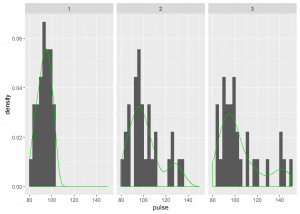Want to share your content on R-bloggers? click here if you have a blog, or here if you don't.One way, two way and n way ANOVA are used to test difference in means when we have one, two and n factor variables. A key assumption when performing these ANOVAs is that the measurements are independent. When we have repeated measures this assumption is violated, so we have to use repeated measures ANOVA. Repeated measures designs occur often in longitudinal studies where we are interested in understanding change over time. For example a medical researcher would be interested in assessing the level of depression before and after a surgery procedure. Repeated measures designs are not limited to longitudinal studies, they can also be used when you have an important variable you would like to repeat measures. For example in a fitness experiment you can repeat your measures at different intensity levels. Repeated measures ANOVA can be considered an extension of the paired t test.

Before diving deeper into repeated measures ANOVA you need to understand terminology used. A subject is a member of the sample under consideration. In our medical study introduced earlier an individual patient is a subject. The within-subjects factor is the variable that identifies how the dependent variable has been repeatedly measured. In our medical study we would measure depression 4 weeks before surgery, 4 weeks after surgery and 8 weeks after surgery. The different conditions when repeated measurements are made are referred to as trials. A between-subjects factor identifies independent groups in the study. For example if we had two different procedures this would be the between subjects factor. These conditions are referred to as groups. Repeated measures analysis requires balance in between-subjects factor. For example subjects in each of surgery procedures need to be equal.

With a repeated measures design we are able to test the following hypotheses.

1. There is no within-subjects main effect
2. There is no between-subjects main effect
3. There is no between subjects interaction effect
4. There is no within subject by between subject interaction effect

There are two assumptions that need to be satisfied when using repeated measures.

1. The dependent variable is normally distributed in each level of the within-subjects factor. Repeated measures analysis is robust to violations of normality with a large sample size which is considered at least 30 subjects. However the accuracy of p values is questionable when the distribution is heavily skewed or thick tailed.
2. The variance across the within subject factor is equal. This is the sphericity assumption. Repeated measures analysis is not robust to this assumption so when there is a violation power decreases and a corresponding increase in probability of a type II error occurs. A Mauchly’s test assesses the null hypothesis variance is equal. The sphericity assumption is only relevant when there are more than 2 levels of the within subjects factor.

When the sphericity assumption is violated we make corrections by adjusting the degrees of freedom. Corrections available are Greenhouse-Geisser, Huynh-Feldt and Lower bound. To make a decision on appropriate correction we use a Greenhouse-Geisser estimate of sphericity (ξ). When ξ < 0.75 or we do not know anything about sphericity the Greenhouse-Geisser is the appropriate correction. When ξ > 0.75 Huynh-Feldt is the appropriate correction.

For this exercise we will use data on pulse rate exer. People were randomized to two diets, three exercise types and pulse was measured at three different time points. For this data time points is the within-subjects factor. The between-subjects factors are diet and exercise type

The solutions to the exercises below can be found here

Exercise 1

Load the data and inspect its structure

Exercise 2

Check for missing values

Exercise 3

Check for balance in between-subjects factor

Exercise 4

Generate descriptive statistics for the sex variable which is a between subjects factor

Exercise 5

Generate descriptive statistics for the treatment level variable which is a between subjects factor

Exercise 6

Generate descriptive statistics for the weeks variable which is the within subjects factor

Exercise 7

Use histograms to assess distribution across within subjects factor.

Exercise 8

Perform a repeated measures analysis with only the within subjects factor

Exercise 9

Perform a repeated measures analysis with the within subjects factor and one between subjects factor

Exercise 10

Perform a repeated measures analysis with the within subjects factor and two between subjects factors